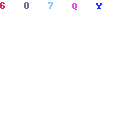# FNCE3004 Semester 2 2017 Assignment

FNCE3004 Semester 2 2017 Assignment
Chapter 6 –International Parity Relationships and Forecasting Foreign Exchange Rates

1. Suppose you observe a spot exchange rate of \$1.50/€. If interest rates are 5% APR in the U.S. and 3% APR in the euro zone, what is the no-arbitrage 1-year forward rate?

 A. €1.5291/\$

 B. \$1.5291/€

 C. €1.4714/\$

 D. \$1.4714/€

Circle the correct answer above and provide the workings to the answer above here:

2. Suppose that the annual interest rate is 2.0 percent in the United States and 4 percent in Germany, and that the spot exchange rate is \$1.60/€ and the forward exchange rate, with one-year maturity, is \$1.58/€. Assume that an arbitrager can borrow up to \$1,000,000 or €625,000

a. Determine whether the interest rate parity is currently holding.
b. If the IRP is not holding, how would you carry out covered interest arbitrage? Show all the steps and determine the arbitrage profit.

 A. \$238.65

 B. \$14,000

 C. \$46,207

 D. \$7,000

Circle the correct answer above and provide the workings to the answer above here:

1. Explain how the IRP will be restored as a result of covered arbitrage activities.

Chapter 07 – Futures and Options on Foreign Exchange

3.    Consider the graph of a call option shown at right. The option is a three-month American call option on €62,500 with a strike price of \$1.50 = €1.00 and an option premium of \$3,125.

i)              What are the values of A, B, and C, respectively?

 A. A = -\$3,125 (or -\$.05 depending on your scale); B = \$1.50; C = \$1.55

 B. A = -€3,750 (or -€.06 depending on your scale); B = \$1.50; C = \$1.55

 C. A = -\$.05; B = \$1.55; C = \$1.60

D. none of the above

ii) Suppose that spot rate on the expiration date is \$1.70/€.  What is the gain/loss?

iii) Suppose that the spot rate on the expiration date is \$1.53/€.  What is the gain/loss?
Solutions: (Student should be able to solve this after studying Ex 7.3 p.g 183)
Circle the correct answer to part i) above and provide the workings to parts i), ii) and iii below:

4.    Today’s settlement price on a Chicago Mercantile Exchange (CME) Yen futures contract is \$0.8011/¥100. Your margin account currently has a balance of \$2,000. The next three days’ settlement prices are \$0.8057/¥100, \$0.7996/¥100, and \$0.7985/¥100. (The contractual size of one CME Yen contract is ¥12,500,000). If you have a long position in one futures contract, the changes in the margin account from daily marking-to-market, will result in the balance of the margin account after the third day to be

 A. \$1,425.

 B. \$1,675.

 C. \$2,000.

 D. \$3,425.

Circle the correct answer above and provide the workings to the answer above here:

Chapter 08 – Management of Transaction Exposure

5. Your firm is a U.K.-based exporter of bicycles. You have sold an order to a French firm for €1,000,000 worth of bicycles. Payment from the French firm (in euro) is due in 12 months. Use a money market hedge to redenominate this one-year receivable into a pound-denominated receivable with a one-year maturity.
The following were computed without rounding. Select the answer closest to yours.

 A. £803,721.49

 B. €800,000

 C. £780,312.13

 D. £72,352.94

Circle the correct answer above and provide the workings to the answer above here:

Chapter 11 – International Banking and Money Market

6. You entered in to a 3 × 6 forward rate agreement that obliged you to borrow \$10,000,000 at 3%. Suppose at the maturity of the FRA, the correct interest rate is 3½%. Clearly you are better off since you have the ability to borrow \$10,000,000 for 3 months at 3% instead of 3½%. What is the payoff at the maturity of the FRA?

 A. Net payment of \$12,391.57 to you

 B. Net payment of \$12,500 to you

 C. Net payment of \$50,000 to you

 D. Net payment of \$48,309.18 to you

Circle the correct answer above and provide the workings to the answer above here: